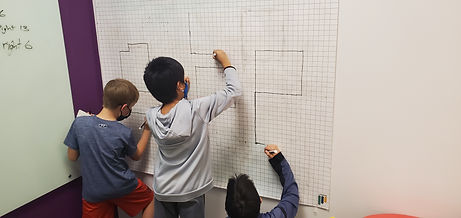Mr. Jake

Target 1​

Lesson Type:

New

Geometry

:

Symmetry

Create symmetrical figures (using understandings that a figure does not change in a symmetrical transformation).

1:

Define translational symmetry.

2:

Understand a translation as a movement (shift or slide) of a figure in a specified direction through a specified distance without any rotation or reflection.

3:

Identify whether a figure has undergone a reflectional, rotational, or translational change.

7th

Vocabulary:

Translate

Activities:

In geometry, to translate a geometric figure is to move it from one place to another without rotating it.

Translated objects on the coordinate plane

Determined if 2 shapes were translations of each otherHome Exploration

Guiding Questions:Absent Students:

Target 2

:

1:

Understand that an exponent indicated the number of times that a base is multiplied by itself.

2:

Write the expanded format for a given exponent (repeating multiplication equation that is equivalent to a given exponent).

3:

Write an exponent that is equivalent to the expanded format (a repeating multiplication equation).

4:

Evaluate expressions containing positive exponents.

7th

Vocabulary:

Exponent, Base, Power

Activities:

Solved equations involving exponents

Wrote the expanded versions of exponentsHome Exploration

Guiding Questions:Target 3

:

Vocabulary:

Activities:Home Exploration